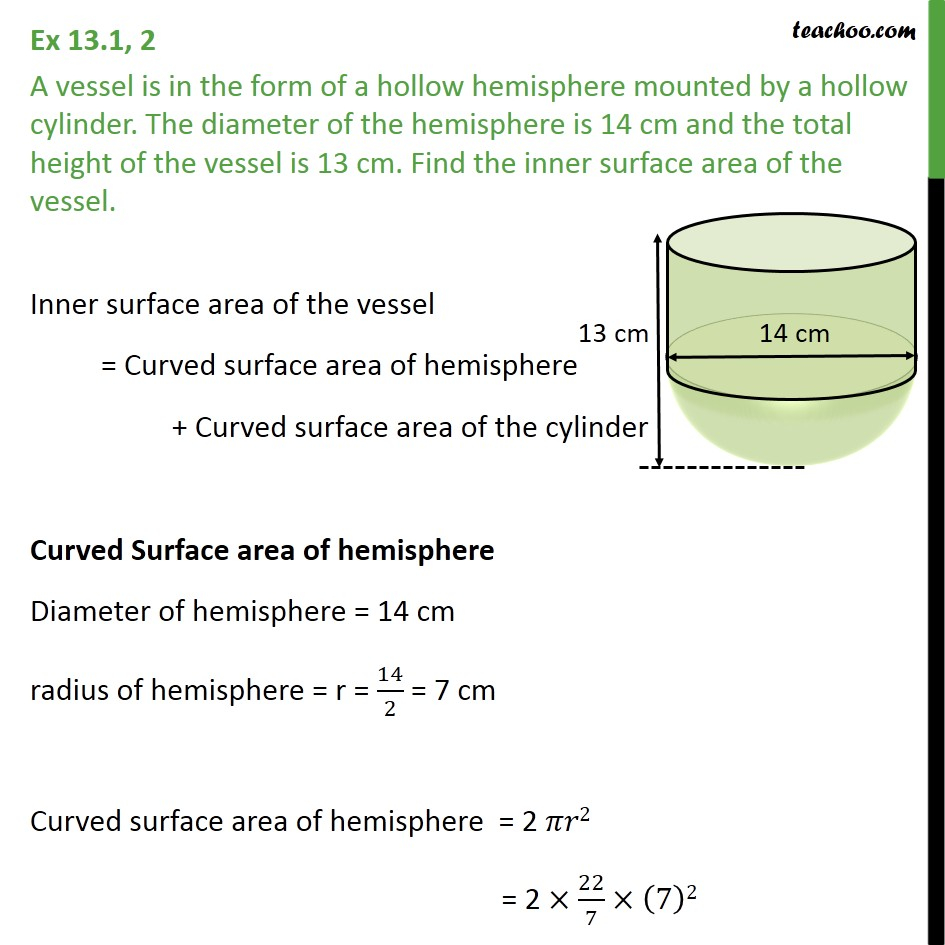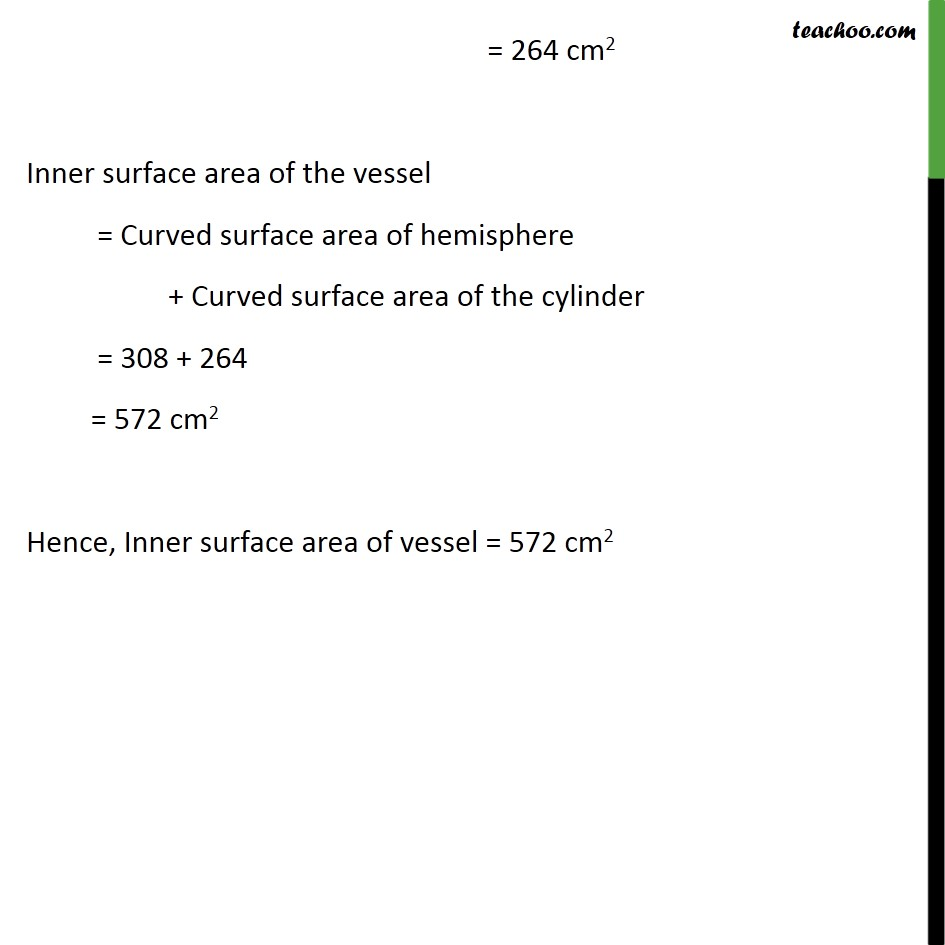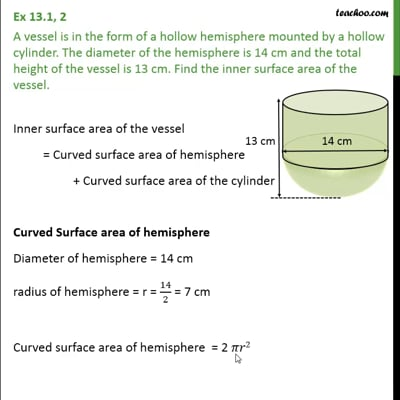Ex 13.1

Chapter 13 Class 10 Surface Areas and Volumes
Serial order wiseThis video is only available for Teachoo black users

Maths Crash Course - Live lectures + all videos + Real time Doubt solving!

### Transcript

Ex 13.1, 2 A vessel is in the form of a hollow hemisphere mounted by a hollow cylinder. The diameter of the hemisphere is 14 cm and the total height of the vessel is 13 cm. Find the inner surface area of the vessel. Inner surface area of the vessel = Curved surface area of hemisphere + Curved surface area of the cylinder Curved Surface area of hemisphere Diameter of hemisphere = 14 cm radius of hemisphere = r = 14/2 = 7 cm Curved surface area of hemisphere = 2 𝜋𝑟2 = 2 ×22/7×(7)2 = 2×22/7×7×7 = 2×22×7 = 308 cm2 Curved surface area of cylinder Curved Surface area of cylinder = 2𝜋𝑟ℎ Radius of cylinder = radius of hemisphere = r = 7 cm Height of cylinder = Total height – Radius of hemisphere = 13 – 7 = 6 cm Curved Surface area of the cylinder = 2𝜋𝑟ℎ = 2×22/7×7×6 = 2 ×22 ×6 = 264 cm2 Inner surface area of the vessel = Curved surface area of hemisphere + Curved surface area of the cylinder = 308 + 264 = 572 cm2 Hence, Inner surface area of vessel = 572 cm2